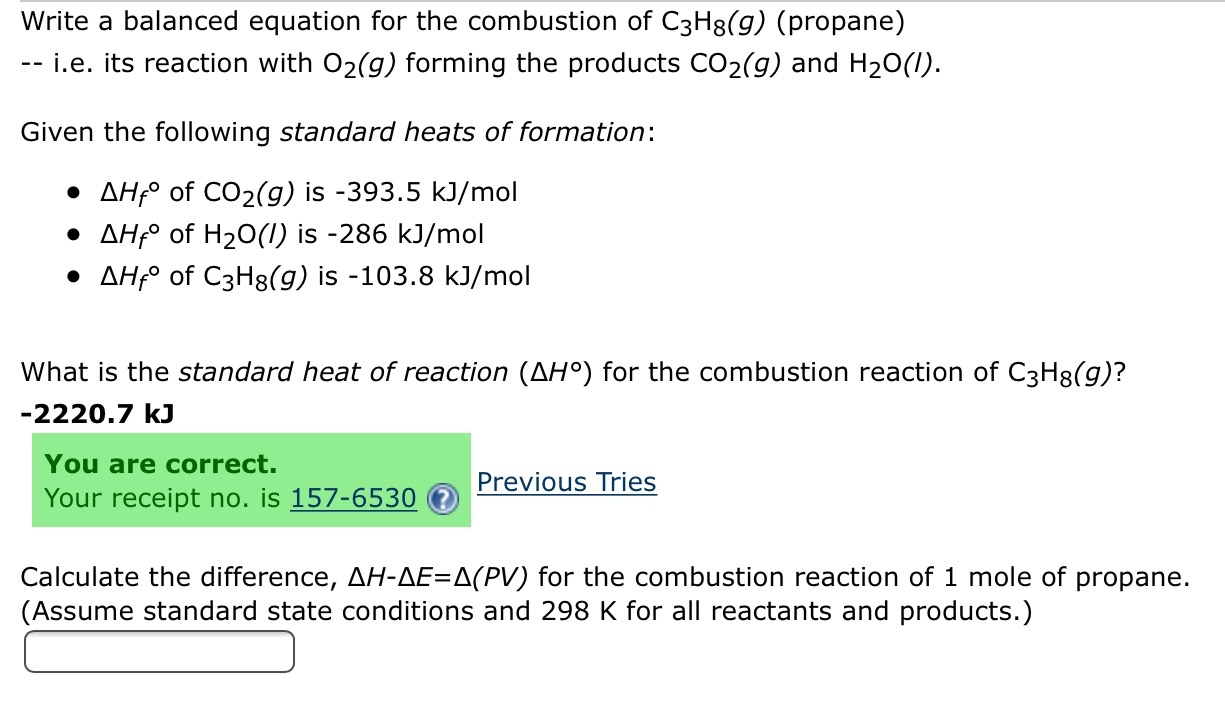# Write a balanced equation for the combustion of C3H8(g) (propane)-i.e. its reaction with O2(g) forming the products CO2(g) and H20()Given the following standard heats of formationAHf of CO2(g) is -393.5 kJ/molAHfo of H2O(I) is -286 kJ/molAHfo of C3H8(g) is -103.8 kJ/molWhat is the standard heat of reaction (AH°) for the combustion reaction of C3H3(g)?-2220.7 kJYou are correct.Previous TriesYour receipt no. is 157-6530Calculate the difference, AH-AE=A(PV) for the combustion reaction of 1 mole of propane.(Assume standard state conditions and 298 K for all reactants and products.)

Questionhelp_outlineImage TranscriptioncloseWrite a balanced equation for the combustion of C3H8(g) (propane) -i.e. its reaction with O2(g) forming the products CO2(g) and H20() Given the following standard heats of formation AHf of CO2(g) is -393.5 kJ/mol AHfo of H2O(I) is -286 kJ/mol AHfo of C3H8(g) is -103.8 kJ/mol What is the standard heat of reaction (AH°) for the combustion reaction of C3H3(g)? -2220.7 kJ You are correct. Previous Tries Your receipt no. is 157-6530 Calculate the difference, AH-AE=A(PV) for the combustion reaction of 1 mole of propane. (Assume standard state conditions and 298 K for all reactants and products.) fullscreen
check_circleExpert Solution
Step 1

The balanced equation is:

Step 2

Enthalpy of combu...

### Want to see the full answer?

See Solution

#### Want to see this answer and more?

Solutions are written by subject experts who are available 24/7. Questions are typically answered within 1 hour*

See Solution
*Response times may vary by subject and question
Tagged in

### General Chemistry